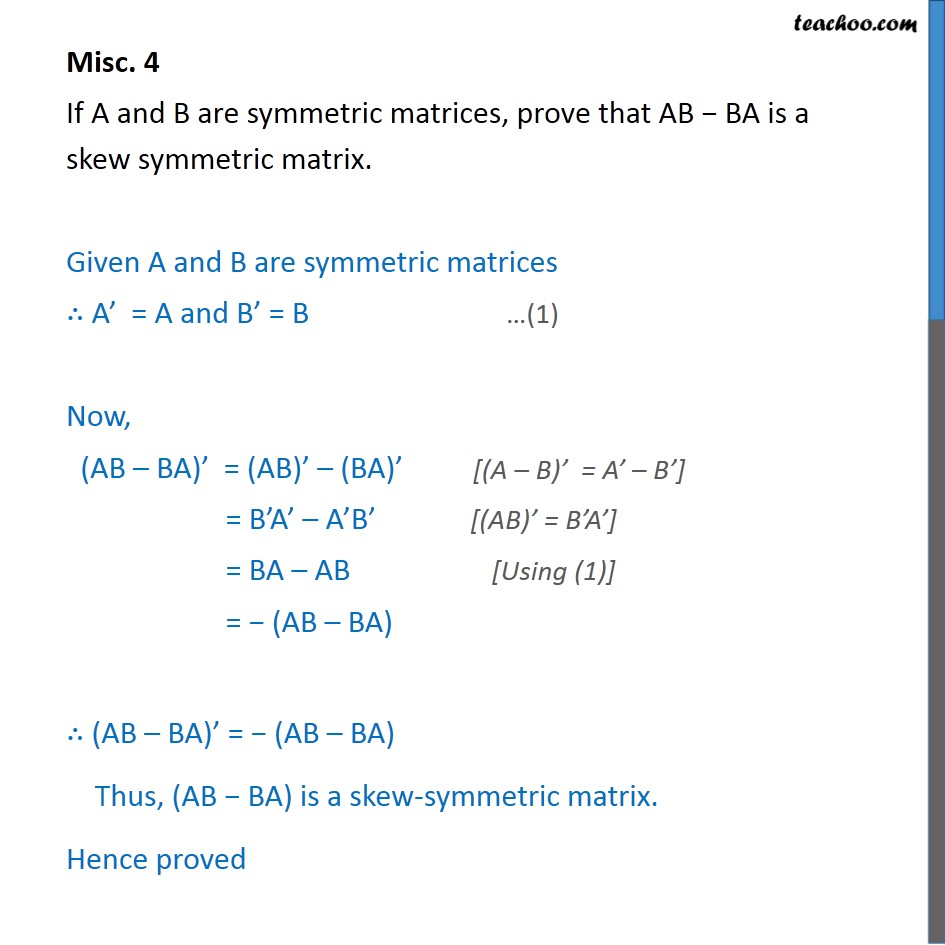1. Chapter 3 Class 12 Matrices
2. Concept wise
3. Proof using property of transpose

Transcript

Misc. 4 If A and B are symmetric matrices, prove that AB − BA is a skew symmetric matrix. Given A and B are symmetric matrices ∴ A’ = A and B’ = B Now, (AB – BA)’ = (AB)’ – (BA)’ = B’A’ – A’B’ = BA – AB = − (AB – BA) ∴ (AB – BA)’ = − (AB – BA) Thus, (AB − BA) is a skew-symmetric matrix. Hence proved

Proof using property of transpose

About the AuthorDavneet Singh
Davneet Singh is a graduate from Indian Institute of Technology, Kanpur. He has been teaching from the past 10 years. He provides courses for Maths and Science at Teachoo.